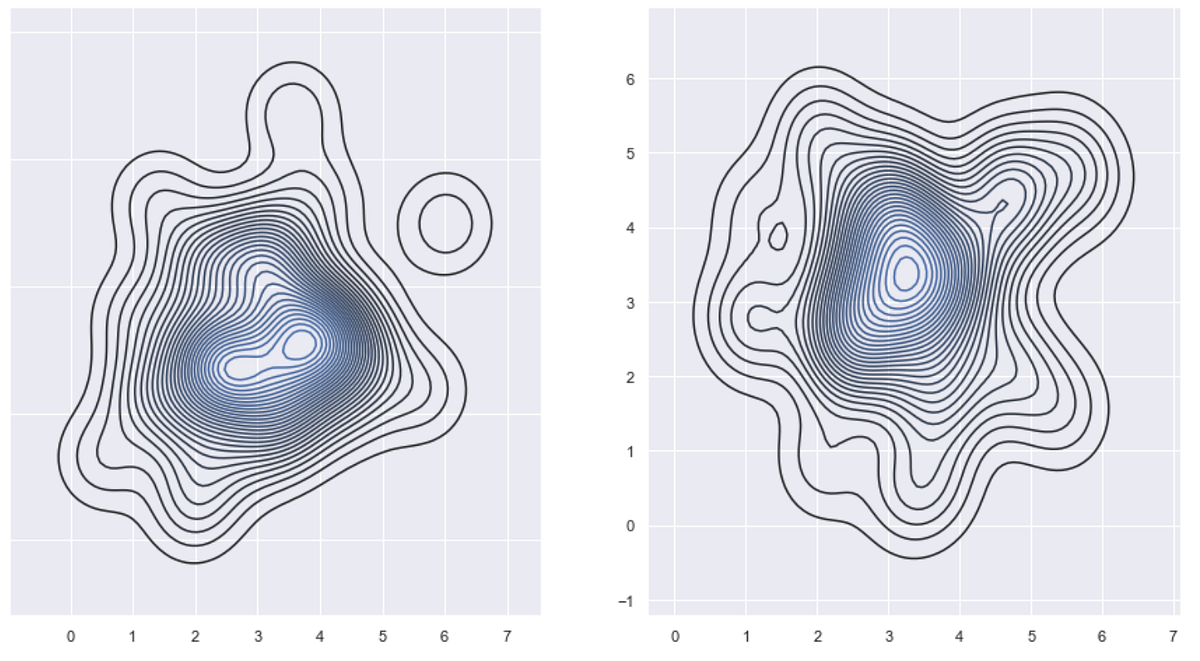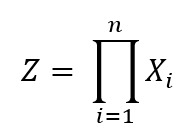# Anomaly detection in monitored systems (part 3)Learning how Gaussian distributions and the properties they have can help us perform anomaly detection on monitored data. In the second article of this series, we learned a bit more about the Gaussian function, and we then built a working anomaly detection system using its properties. In this final part, we will be dealing with the multivariate case, as promised in part 1 of the series.

In the second article of this series, we learned a bit more about the Gaussian function, and we then built a working anomaly detection system using its properties. In this final part, we will be dealing with the multivariate case, as promised in part 1 of the series. Unlike parts 1 and 2, which didn’t require any previous knowledge, this article will require at least basic knowledge of linear algebra (especially working with vectors and matrices).

So what exactly is a multivariate distribution? The “multivariate” part generalizes distributions in one dimension to any finite number of dimensions. This makes multivariate distributions incredibly useful in modeling data associated with many monitored parameters of a given device, all at once. The method by which we extend our definition to higher dimensions is by something called a “random vector”. A vector X = (X1, X2,…, Xn) is called a random vector if Xk is a random variable for every 1≤k≤n. A random vector is considered to be normally distributed if, in addition to the property above, any linear combination of its random variables is also a normally distributed random variable.

How do we interpret such reading to give us a classification of anomalies in the behaviour of monitored devices? The simplest way is perhaps to multiply the random variables with each other. Because a random variable monitors output over time, we can treat our random variables as vectors whose values are just ordinary numbers from a given field. We can then multiply them element-wise to give us what is called a product distribution:Z is the product distribution of all random variables in the random vector X

We can the establish the mean and the standard deviation of our product distribution, and use them to calculate the probability of a sample being anomalous, with respect to the product distribution Z: Whenever we read a new group of values from our monitored device, we construct a vector z = (z1, z2,…,zn) where zi denotes the latest value of the i-th monitored parameter. We then calculate the probability of z being an anomaly by multiplying the independent values with each other:

## HTML entity encoder decoder Online

Online Data Science Training in Noida at CETPA, best institute in India for Data Science Online Course and Certification. Call now at 9911417779 to avail 50% discount.

## Data Science Course in Dallas

Become a data analysis expert using the R programming language in this [data science](https://360digitmg.com/usa/data-science-using-python-and-r-programming-in-dallas "data science") certification training in Dallas, TX. You will master data...

## 50 Data Science Jobs That Opened Just Last Week

Data Science and Analytics market evolves to adapt to the constantly changing economic and business environments. Our latest survey report suggests that as the overall Data Science and Analytics market evolves to adapt to the constantly changing economic and business environments, data scientists and AI practitioners should be aware of the skills and tools that the broader community is working on. A good grip in these skills will further help data science enthusiasts to get the best jobs that various industries in their data science functions are offering.

## Why You Should Learn Statistical Programming

It generally covers statistics, mathematics, physics, economics, business, and management. Here, we’ll go to different reasons for those undergraduates to learn statistical programming.

## Statistics for Data Science

Statistics for Data Science and Machine Learning Engineer. I’ll try to teach you just enough to be dangerous, and pique your interest just enough that you’ll go off and learn more.